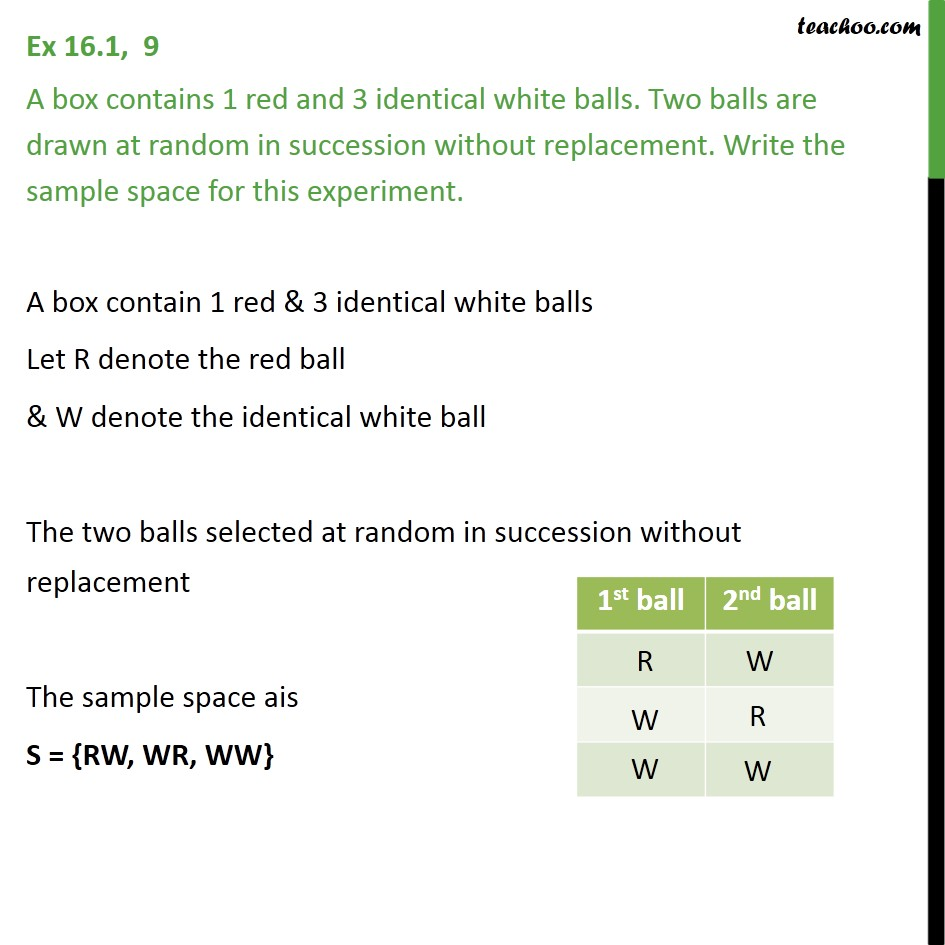1. Chapter 16 Class 11 Probability (Term 2)
2. Serial order wise
3. Ex 16.1

Transcript

Ex 16.1, 9 A box contains 1 red and 3 identical white balls. Two balls are drawn at random in succession without replacement. Write the sample space for this experiment. A box contain 1 red & 3 identical white balls Let R denote the red ball & W denote the identical white ball The two balls selected at random in succession without replacement The sample space ais S = {RW, WR, WW}

Ex 16.1

Chapter 16 Class 11 Probability (Term 2)
Serial order wise